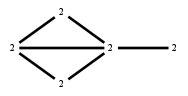# The Markov Bases Database

## G40g_bin

The binary graphical model of G40.

It is a hierarchical model of 5 variables. The dimension of the model is 13.The cardinality of the statespace is 32.

### Properties of the Markov basis

 Markov degree 2 68

 degree 2 68

The model has the following properties:

• All variables are binary.
• It is a graphical model.
• The semigroup is normal.### FILES

 Markov basis: sufficient statistics matrix: G40g_bin.mar (6.45 kb) G40g_bin.mat (1.28 kb) G40g_bin.mod (37 b) G40g_bin.tar.gz (7.00 kb)